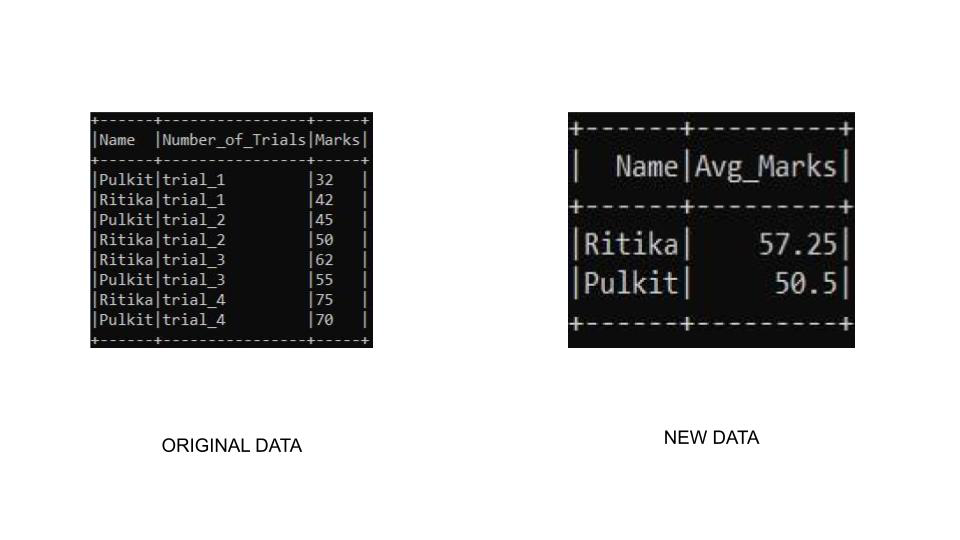Related Articles

# PySpark – GroupBy and sort DataFrame in descending order

• Last Updated : 23 May, 2021

In this article, we will discuss how to groupby PySpark DataFrame and then sort it in descending order.

### Methods Used

• groupBy(): The groupBy() function in pyspark is used for identical grouping data on DataFrame while performing an aggregate function on the grouped data.

Syntax: DataFrame.groupBy(*cols)

Parameters:

• cols→ Columns by which we need to group data
• sort(): The sort() function is used to sort one or more columns. By default, it sorts by ascending order.

Syntax: sort(*cols, ascending=True)

Parameters:

• cols→ Columns by which sorting is needed to be performed.
• PySpark DataFrame also provides orderBy() function that sorts one or more columns. By default, it orders by ascending.

Syntax: orderBy(*cols, ascending=True)

Parameters:

• cols→ Columns by which sorting is needed to be performed.
• ascending→ Boolean value to say that sorting is to be done in ascending order

Example 1: In this example, we are going to group the dataframe by name and aggregate marks. We will sort the table using the sort() function in which we will access the column using the col() function and desc() function to sort it in descending order.

## Python3

 `# import the required modules``from` `pyspark.sql ``import` `SparkSession``from` `pyspark.sql.functions ``import` `avg, col, desc`` ` `# Start spark session``spark ``=` `SparkSession.builder.appName(``"GeeksForGeeks"``).getOrCreate()`` ` `# Define sample data``simpleData ``=` `[(``"Pulkit"``,``"trial_1"``,``32``),``    ``(``"Ritika"``,``"trial_1"``,``42``),``    ``(``"Pulkit"``,``"trial_2"``,``45``),``    ``(``"Ritika"``,``"trial_2"``,``50``),``    ``(``"Ritika"``,``"trial_3"``,``62``),``    ``(``"Pulkit"``,``"trial_3"``,``55``),``    ``(``"Ritika"``,``"trial_4"``,``75``),``    ``(``"Pulkit"``,``"trial_4"``,``70``)``  ``]`` ` `# define the schema``schema ``=` `[``"Name"``,``"Number_of_Trials"``,``"Marks"``]`` ` `# create a dataframe``df ``=` `spark.createDataFrame(data``=``simpleData, schema ``=` `schema)`` ` `# group by name and aggrigate using``# average marks sort the column using``# col and desc() function``df.groupBy(``"Name"``) \``  ``.agg(avg(``"Marks"``).alias(``"Avg_Marks"``)) \``  ``.sort(col(``"Avg_Marks"``).desc()) \``  ``.show()`` ` `# stop spark session``spark.stop()`

Output:Example 2: In this example, we are going to group the dataframe by name and aggregate marks. We will sort the table using the sort() function in which we will access the column within the desc() function to sort it in descending order.

## Python3

 `# import the required modules``from` `pyspark.sql ``import` `SparkSession``from` `pyspark.sql.functions ``import` `avg, col, desc`` ` `# Start spark session``spark ``=` `SparkSession.builder.appName(``"Student_Info"``).getOrCreate()`` ` `# sample dataset``simpleData ``=` `[(``"Pulkit"``,``"trial_1"``,``32``),``    ``(``"Ritika"``,``"trial_1"``,``42``),``    ``(``"Pulkit"``,``"trial_2"``,``45``),``    ``(``"Ritika"``,``"trial_2"``,``50``),``    ``(``"Ritika"``,``"trial_3"``,``62``),``    ``(``"Pulkit"``,``"trial_3"``,``55``),``    ``(``"Ritika"``,``"trial_4"``,``75``),``    ``(``"Pulkit"``,``"trial_4"``,``70``)``  ``]`` ` `# define the schema to be used``schema ``=` `[``"Name"``,``"Number_of_Trials"``,``"Marks"``]`` ` `# create the dataframe``df ``=` `spark.createDataFrame(data``=``simpleData, schema ``=` `schema)`` ` `# perform groupby operation on name table``# aggrigate marks and give it a new name``# sort in descending order by avg_marks``df.groupBy(``"Name"``) \``  ``.agg(avg(``"Marks"``).alias(``"Avg_Marks"``)) \``  ``.sort(desc(``"Avg_Marks"``)) \``  ``.show()`` ` `# stop sparks session``spark.stop()`

Output:Example 3: In this example, we are going to group the dataframe by name and aggregate marks. We will sort the table using the orderBy() function in which we will pass ascending parameter as False to sort the data in descending order.

## Python3

 `# import required modules``from` `pyspark.sql ``import` `SparkSession``from` `pyspark.sql.functions ``import` `avg, col, desc`` ` `# Start spark session``spark ``=` `SparkSession.builder.appName(``"Student_Info"``).getOrCreate()`` ` `# sample dataset``simpleData ``=` `[(``"Pulkit"``,``"trial_1"``,``32``),``    ``(``"Ritika"``,``"trial_1"``,``42``),``    ``(``"Pulkit"``,``"trial_2"``,``45``),``    ``(``"Ritika"``,``"trial_2"``,``50``),``    ``(``"Ritika"``,``"trial_3"``,``62``),``    ``(``"Pulkit"``,``"trial_3"``,``55``),``    ``(``"Ritika"``,``"trial_4"``,``75``),``    ``(``"Pulkit"``,``"trial_4"``,``70``)``  ``]`` ` `# define the schema``schema ``=` `[``"Name"``,``"Number_of_Trials"``,``"Marks"``]`` ` `# create a dataframe``df ``=` `spark.createDataFrame(data``=``simpleData, schema ``=` `schema)`` ` `df.groupBy(``"Name"``)\``    ``.agg(avg(``"Marks"``).alias(``"Avg_Marks"``))\``    ``.orderBy(``"Avg_Marks"``, ascending``=``False``)\``    ``.show()`` ` `# stop sparks session``spark.stop()`

Output:Attention geek! Strengthen your foundations with the Python Programming Foundation Course and learn the basics.

To begin with, your interview preparations Enhance your Data Structures concepts with the Python DS Course. And to begin with your Machine Learning Journey, join the Machine Learning – Basic Level Course

My Personal Notes arrow_drop_up